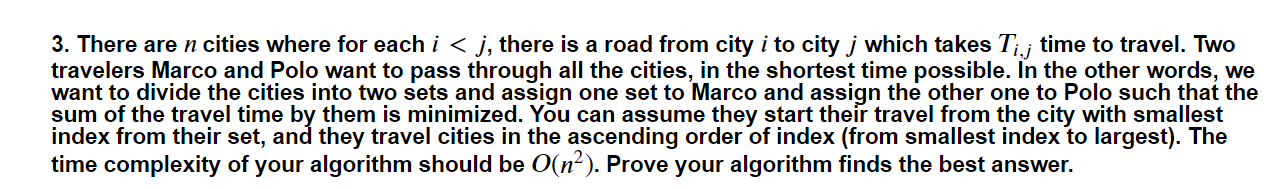cost[i][j]表示从城市i到j的时间;
prefix[i]表示cost+cost+...cost[i-1][i];
dp[i][j]表示遍历城市i,i+1,...j的最优解并且j城市由Macro遍历;
dp[i][j]表示遍历城市i,i+1,...j的最优解并且j城市由Polo遍历;

dp[i][j] = min(dp[i][k] + prefix[i] - prefix[k], dp[i][k] + cost[A[k]][k+1] + prefix[i]- prefix[k+1]);
A[k]表示当取得dp[i][k]时Polo所在城市， A[k] < k;
dp[i][j] = min(dp[i][k] + prefix[i] - prefix[k], dp[i][k] + cost[B[k]][k+1] + prefix[i]- prefix[k+1]);
B[k]表示当取得dp[i][k]时Macro所在城市，B[k] < k;

。。。又刷到了你的帖子，是缘分吗。。讲道理我又没有体会到这道题的dp或者分治思想，不太好意思写回答。。不过既然是作业提供一个解题思路先完成了先吧